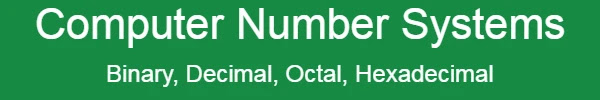# Computer Number Systems | Binary, Decimal, Octal

The number system is a method of writing any number, if the method is understood in the easy language then it is a rule (system). If it is said in computer language then it is a process of showing numbers on the computer. The process of displaying numbers on a computer is called a number system. We use the decimal number system (1, 2, 3, 4, 5, 6, 7, 8, and 9) while the computer works on the binary number system (0 and 1).

## Types of Computer Number System

Mainly Computer Number System is defined based on the number of digits present in them. Computer Number System consists of the following four types:-

1. Decimal Number System
2. Binary Number System
3. Octal Number System### Decimal Number System

This number system is the number used in our daily life. Human uses decimal numbers in their daily life. These numbers are 10 in number, hence they are called decimal and their base is also 10. These numbers 0, 1, 2, 3, 4, 5, 6, 7, 8, and 9 are used to represent any decimal number, where each number has its own absolute value and a place value.

Example:-

6352 = 6000+300+50+2

= 6×1000 + 3×100 + 5×10 + 2

= 6×10+ 3×10+ 5×10+ 2×100

### Binary Number System

The binary number system has two digits 0 or 1 due to the ON/OFF concept of the electrical device. Computer work with two signals i.e pulse and no-pulse state is known as binary 0 and the no-pulse state is known as binary. For this, the binary system has its radix as 2.

Example:-

1011 = 1×2+ 0×2+ 1×2+ 1×20

= 8 + 0 + 2 + 1

= 11 (Decimal)

### Octal Number System

The octal number system consists of only 8 digits from 0 to 7. These digits are 0, 1, 2, 3, 4, 5, 6, and 7. The base or radix of this number system is 8.

Example:- (2532)8